#### You may also like### Diophantine N-tuples

Can you explain why a sequence of operations always gives you perfect squares?### DOTS Division

Take any pair of two digit numbers x=ab and y=cd where, without loss of generality, ab > cd . Form two 4 digit numbers r=abcd and s=cdab and calculate: {r^2 - s^2} /{x^2 - y^2}.### Sixational

The nth term of a sequence is given by the formula n^3 + 11n . Find the first four terms of the sequence given by this formula and the first term of the sequence which is bigger than one million. Prove that all terms of the sequence are divisible by 6.

# Hike and Hitch

##### Age 14 to 16Challenge Level

The only completely correct solution to this question came, with a very nice diagram, from Vassil Vassilev, age 14, Lawnswood High School, Leeds.

If nobody hiked, the car would need two return journeys plus one single journey, making a total distance of $5\times 60$ miles and hence a total time of $(5\times 60)/40 = 7\,{\rm hrs}\ 30\,{\rm mins}$. You have to find out how much time is saved by the students hiking whenever they are not in the car.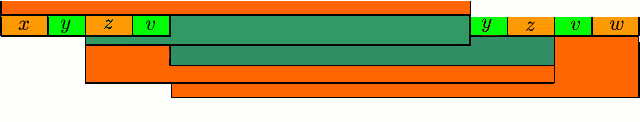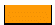- The distance 10 children moved when the car was going towards the destination point.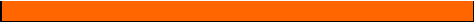- The distance the car moved towards the destination point.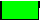- The distance 15 children moved when the car was going back.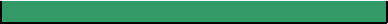- The distance the car moved back to pick up the rest of the children.

You could work out all the distances but that involves a lot of equations. It is best to focus on the time taken. Let $T$ hours be the total time in which 5 people (plus the driver) are in the car going towards the destination point, and$t$ hours be the total time in which the driver only is in the car going back. We assume that the optimal solution is such that all of the people reach the destination at the same time , that is after$T+t$ hours.

In total, the fifteen people cover $15\times 60$ ($=900$ miles, and this is made up from the miles hitched in the car, and miles hiked on foot. The number of person-miles hitched in the car is$5\times 40T$ , and the number of person-miles hiked is

$$10\times 4T + 15 \times 4t,$$

because 10 people are hiking for $T$ hours (when 5 other people are in the car), and all 15 people are hiking when only the driver is in the car. Thus

$$5\times 40T + (10\times 4T + 15 \times 4t) = 900.$$

This simplifies to give

$$12T + 3t = 45.$$

The car spends $T$ hours travelling forwards, and$t$ hours travelling back towards the start. It therefore travels forwards a distance of$40T$, and backwards a distance of $40t$. Hence$40(T-t)=60$ , or

$$2T-2t=3.$$

Solving these two simultaneous equations for $T$ and $t$, we get$T = 1\,{\rm hr}\ 48\,{\rm mins}$, $t = 3\,{\rm hr}\ 18\,{\rm mins}$, and the total time is $5\,{\rm hr}\ 6\,{\rm mins}$.

As the time would be $7\,{\rm hr}\ 30\,{\rm mins}$ if everyone did the whole journey by car, the time saved is $2\,{\rm hrs}\ 24\,{\rm mins}$.